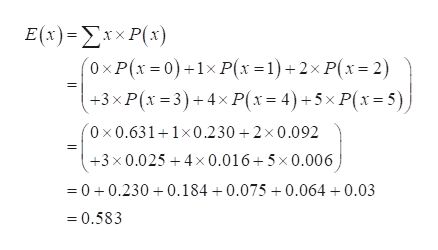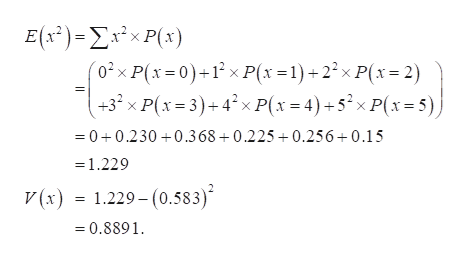# Dogs012345 Probability0.6310.2300.0920.0250.0160.006​(a) Find the​ mean, variance, and standard deviation of the probability distribution.

Question
21 views
 Dogs Probability 0 1 2 3 4 5 0.631 0.23 0.092 0.025 0.016 0.006
​(a) Find the​ mean, variance, and standard deviation of the probability distribution.
check_circle

Step 1

Mean:

The general formula to obtain the expected value (mean) of a discrete random variable is,

E(X) = ∑X*P(X)

The mean of the variable x is obtained as 0.583 from the calculation given below:help_outlineImage TranscriptioncloseE(x) -Συχ P(x) '0xP(x0)1xP(x =1)+2x P(x 2) +3x P(x 3)+4x P(x= 4) +5xP(x= 5) 0x0.6311x0.2302 x 0.092 +3 x0.025 4x 0.016 5x0.006 =0 0.2300.184 +0.075 +0.064 +0.03 0.583 fullscreen
Step 2

Variance:

The general formula to obtain the expected value (mean) of a discrete random variable is,

V(X) = ∑X2*P(...help_outlineImage TranscriptioncloseE(s)-ΣέxP's)| '02x P(x 0)+1 x P(x 1)22x P(x 2) +3 x P(x=3)+4?xP(x4)+5xP(x=5) 0+0.230 0.368+0.225+0.256+0.15 =1.229 V(x) = 1.229- (0.583) =0.8891 fullscreen

### Want to see the full answer?

See Solution

#### Want to see this answer and more?

Solutions are written by subject experts who are available 24/7. Questions are typically answered within 1 hour.*

See Solution
*Response times may vary by subject and question.
Tagged in

### Hypothesis Testing